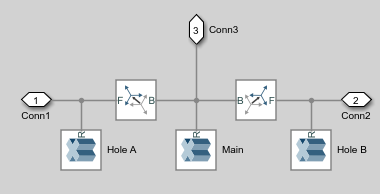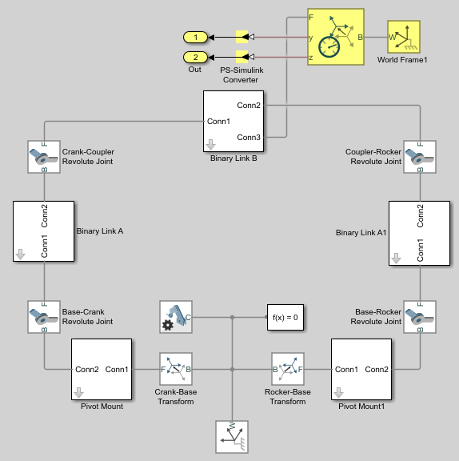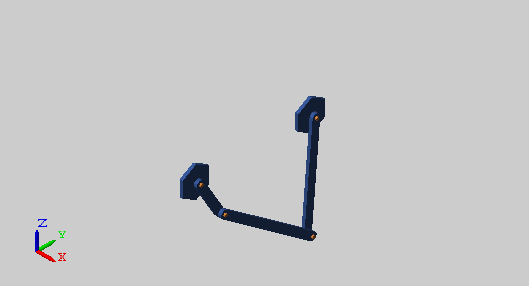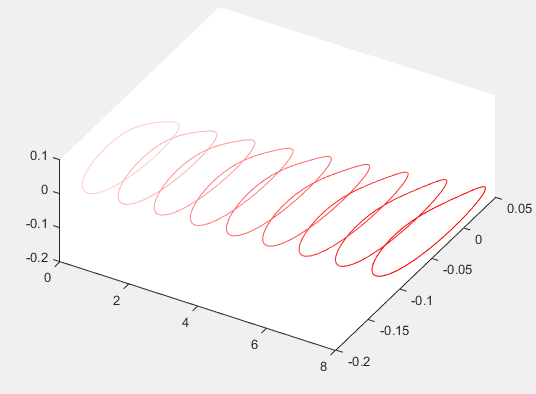## Analyze Motion at Various Parameter Values

This example shows how to use a MATLAB® script to study the dynamic behaviours of a four-bar model at various coupler lengths. The script uses the coupler motion coordinates, obtained using a Transform Sensor block, to plot the resulting coupler curve at each value of the coupler length. For more information about multibody dynamics simulations, see Multibody Dynamics.### Build Model

1. At the MATLAB command prompt, enter `openExample("sm/DocFourBarLinkageModelExample")`. A four-bar model opens up. For instructions on how to create this model, see Model a Closed-Loop Kinematic Chain.

2. Under the mask of the Binary Link B block, connect a third Outport block as shown in the figure. You can add an Outport block by copying and pasting Conn1 or Conn2. The new block identifies the frame whose trajectory you plot in this tutorial.3. Add the following blocks to the model. During simulation, the Transform Sensor block computes and outputs the coupler trajectory with respect to the world frame.

LibraryBlockQuantity
Frames and TransformsWorld Frame1
Frames and TransformsTransform Sensor1

4. In the Transform Sensor block dialog box, select these variables:

• Translation > Y

• Translation > Z

The block exposes frame ports y and z, through which it outputs the coupler trajectory coordinates.

5. Connect the blocks as shown in the figure. Be sure to flip the Transform Sensor block so that its base frame port, labeled B, connects to the World Frame block.### Specify Block Parameters

1. In the Mechanism Configuration block, change Uniform Gravity to `None`.

2. In the Base-Crank Revolute Joint block, specify the following velocity state targets. The targets provide an adequate source of motion for the purposes of this tutorial.

• Select State Targets > Specify Velocity.

• In State Targets > Specify Velocity > Value, enter `2` rev/s.

• Deselect State Target > Specify Position.

3. Specify the following link lengths. The coupler link length is parameterized in terms of a MATLAB variable, `LCoupler`, enabling you change its value iteratively using a simple MATLAB script.

BlockParameterValue

4. Save the model in a convenient folder, naming it `DocFourBarLinkageModelExampleMsensing`.

### Create Simulation Script

Create a MATLAB script to iteratively run simulation at various coupler link lengths:

1. On the MATLAB toolstrip, click New Script.

2. In the script, enter the following code:

```% Run simulation nine times, each time % increasing coupler length by 1 cm. % The original coupler length is 20 cm. for i = (0:8); LCoupler = 20+i; % Simulate model at the current coupler link length (LCoupler), % saving the Outport block data into variables y and z. [~, ~, y, z] = sim('DocFourBarLinkageModelExampleMsensing'); % Plot the [y, z] coordinates of each coupler curve % on the x = i plane. i corresponds to the simulation run number. x = zeros(size(y)) + i; plot3(x, y, z, 'Color', [1 0.8-0.1*i 0.8-0.1*i]); view(30, 60); hold on; end```
The code runs simulation at nine different coupler link lengths. It then plots the trajectory coordinates of the coupler link center frame with respect to the world frame. Coupler link lengths range from 20 cm to 28 cm.

3. Save the script as `simFourBar` in the folder containing the four-bar model.

### Run Simulation Script

Run the `simFourBar` script. In the MATLAB Editor toolstrip, click the Run button or, with the editor active, press F5. Mechanics Explorer opens with a dynamic 3-D view of the four-bar model.Simscape Multibody™ iteratively runs each simulation, adding the resulting coupler link curve to the active plot. The figure shows the final plot.You can use the simple approach shown in this tutorial to analyze model dynamics at various parameter values. For example, you can create a MATLAB script to simulate a crank-slider model at different coupler link lengths, plotting for each simulation run the constraint force acting on the piston.